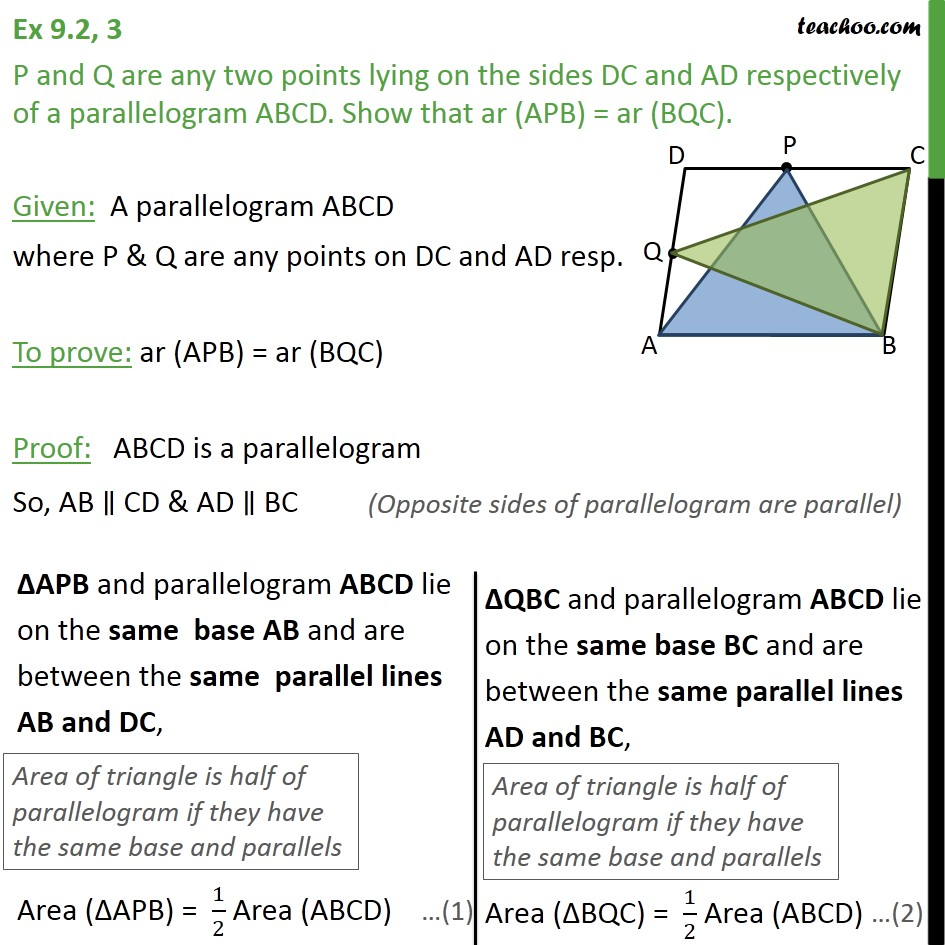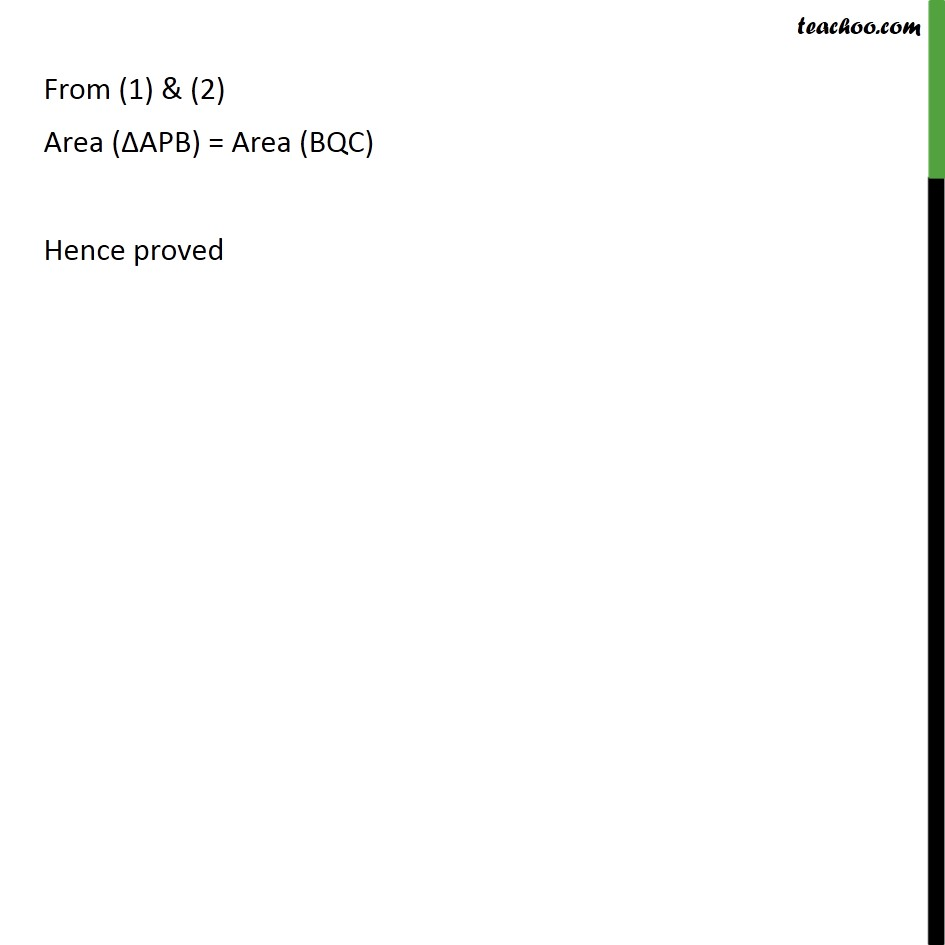Area of Parallelograms

Areas of Parallelograms and Triangles
Serial order wiseLearn in your speed, with individual attention - Teachoo Maths 1-on-1 Class

### Transcript

Question 3 P and Q are any two points lying on the sides DC and AD respectively of a parallelogram ABCD. Show that ar (APB) = ar (BQC). Given: A parallelogram ABCD where P & Q are any points on DC and AD resp. To prove: ar (APB) = ar (BQC) Proof: ABCD is a parallelogram So, AB CD & AD BC From (1) & (2) Area ( APB) = Area (BQC) Hence proved